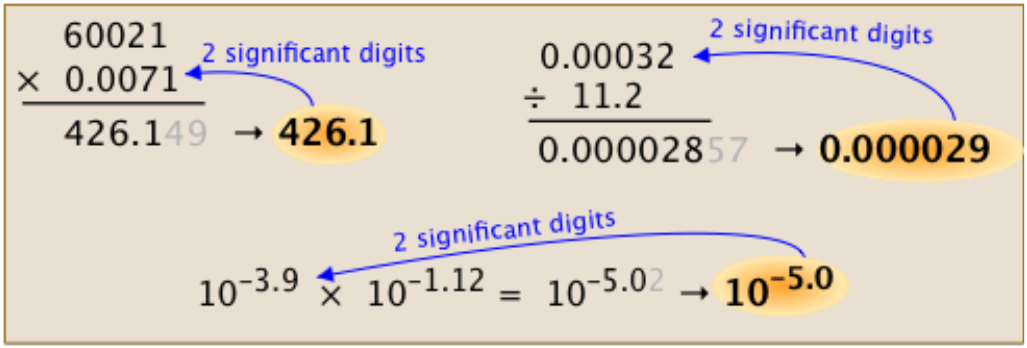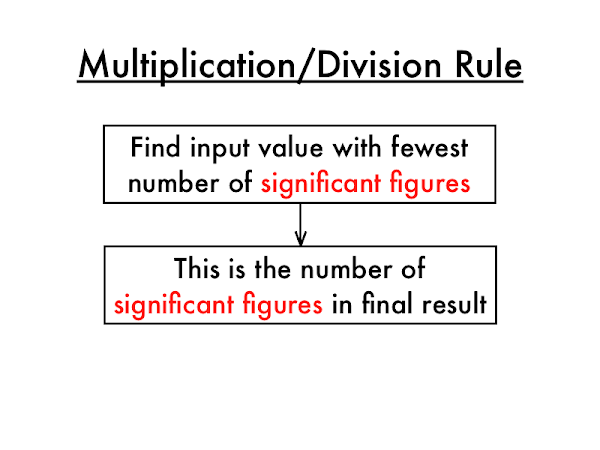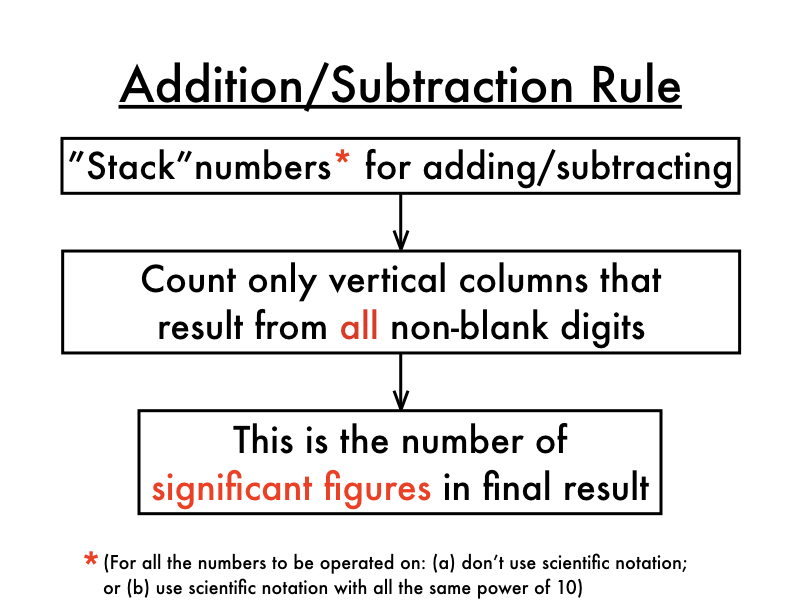Ravela Da Cruz

HS-PS1-3

# Significant Figures in Multiplication and Division Study Guide

When performing mathematical operations on measured values with significant figures (addition, subtraction, multiplication, division), you must follow a set of rules to identify the significant figures for the result.

Table of content

## INTRODUCTION

Calculators🧮 exactly perform what you want them to do, nothing more and nothing less. They can occasionally get out of hand. Suppose you multiply 1.23 by 5.2, you obtain 6.396, a value that ignores the number of significant numbers in either number 🤔. Using a calculator for significant figures gets more complicated.Source

### ROUNDING

We must be able to round numbers correctly before dealing with the technicalities of the procedures for determining the significant digits in a calculated result. Decide how many significant figures a number should have before rounding it. Once you have it, start rounding to that many digits from the left.

If the number immediately to the right of the final significant digit is less than 5, it is ignored, and the last significant digit's value remains unchanged. The last significant digit is incremented by one if the number immediately to its right is larger than or equal to 5.

### MULTIPLICATION AND DIVISION UNCERTAINTY

The density of an object is determined by multiplying its mass by its volume. Consider dividing a mass of 32.46 g by a volume of 10.7 cm3. On a calculator, the result would be:

D = m/V = 37.46 g / 12.7 cm³ = 3.03364486299 g/cm³

The value of the mass measurement contains four significant numbers, but the value of the volume measurement only has three. The answer should be rounded to the same number of significant figures as the measurement with the least number of significant figures in multiplication and division problems.

This rule yields a density of 3.033 g/cm³ with three significant numbers, the same as the volume measurement. The same is the case in the multiplication of significant figures.Source

### USE THE FOLLOWING SIGNIFICANT RULES FOR ADDITION AND SUBTRACTION

• Count the number of significant figures in each integer in the problem's decimal part ONLY.
• In the usual way, add or subtract.
• No more significant figures to the right of the decimal in your final solution than the LEAST number of significant figures in any number in the problem.Source

## CONCLUSION:

• The answer should be rounded to the same number of significant figures as the measurement with the least number of significant figures in multiplication and division problems.
• Count the number of significant figures in each problem just in the decimal portion. In the usual way, add or subtract. No more significant figures to the right of the decimal in your final solution than the LEAST number of significant figures in any number in the problem.

### FAQs:

1. What is the rule for significant figures when multiplying?

Use the following rule for multiplication and division: The number of significant figures in the response is determined by the LEAST number of significant figures in any number in the problem.

2. Do you add significant figures when multiplying?

No, we do not add significant figures when multiplying.

We hope you enjoyed studying this lesson and learned something cool about Significant Figures in Multiplication and Division! Join our Discord community to get any questions you may have answered and to engage with other students just like you! Don't forget to download our App to experience our fun VR classrooms - we promise, it makes studying much more fun! 😎

## SOURCE:

1. Significant Figures in Multiplication and Division. https://www.ck12.org/c/chemistry/significant-figures-in-multiplication-and-division/lesson/Uncertainty-in-Multiplication-and-Division-CHEM/. Accessed 18 Feb 2022.